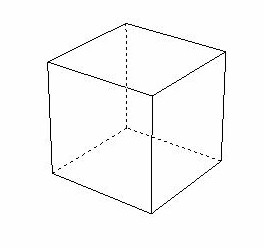```
goal: math of cube rotationHere's a page that has a bunch on cube rotation:
http://www.euclideanspace.com/maths/discrete/groups/categorise/finite/cube/

Let's set up a coordinate system.

Set the cube at the origin. Look down Z at standard X (horizontal), Y
(vertical) plane (right-hand rule).

Let's unfold the cube into 2d

+--------+
|  top   |
+------+--------+-------+------+
| left | front  | right | back |
+------+--------+-------+------+
| bottom |
+--------+

Let's store a cube as:

tlfrbm = 012345 = [ top, left, front, right, back, bottom]

Rotating clockwise about Z (twist from front) CLZ transforms to:
lmftbr

Rotating clockwise about Y (twist from top) CLY transforms to:
tfrblm

Rotating clockwise about X (twist from right) CLX transforms to:
flmrtb

Here's some python code that implements this:

################################
def rotate90( state, direction, axis ):
""">>>> Rotate a cube 90 degrees given:

Set the cube at the origin. Look down Z at standard X (horizontal), Y
(vertical) plane (right-hand rule).

Let's unfold the cube into 2d

+--------+
|  top   |
+------+--------+-------+------+
| left | front  | right | back |
+------+--------+-------+------+
| bottom |
+--------+

Let's store a cube as:

tlfrbm = 012345 = [ top, left, front, right, back, bottom]

- state: string of length 6 = tlfrbm

- direction: = CL/CC = (CL)ockwise or (C)ounter(C)lockwise

- axis: = X/Y/Z in standard cube at the origin. Look down Z at
standard X (horizontal), Y (vertical) plane (right-hand rule).
<<<<
"""

defaultXform = "tlfrbm"
xformToIndex = dict( zip(defaultXform, range(len(defaultXform))))
# {'b': 4, 'f': 2, 'm': 5, 'l': 1, 'r': 3, 't': 0}

xform = "NotSet"

if direction=="CL" and axis=="Z":
# Rotating clockwise about Z (twist from front) CLZ transforms to:
xform = "lmftbr"

if direction=="CL" and axis=="Y":
# Rotating clockwise about Y (twist from top) CLY transforms to:
xform = "tfrblm"

if direction=="CL" and axis=="X":
# Rotating clockwise about X (twist from right) CLX transforms to:
xform = "flmrtb"

newstate = ["x"]*6
for ii in range(6):
newstate[ii] = state[ xformToIndex[xform[ii]] ]

return("".join(newstate))

################################

# Let's try to code out:

print rotate90("tlfrbm", "CL", "X")
# flmrtb
print rotate90("tlfrbm", "CL", "Y")
# tfrblm
print rotate90("tlfrbm", "CL", "Z")
# lmftbr

print rotate90("012345", "CL", "X")
# 215304

# four rotations should be the same
st = "tlfrbm"
for ii in range(4):
newst = rotate90(st, "CL", "X")
print "ii", ii, "st", st, "newst", newst
st = newst

output = """
ii 0 st tlfrbm newst flmrtb
ii 1 st flmrtb newst mlbrft
ii 2 st mlbrft newst bltrmf
ii 3 st bltrmf newst tlfrbm

Yes, back to original state for Clockwise rotation about X. left and
right are invariant.
"""